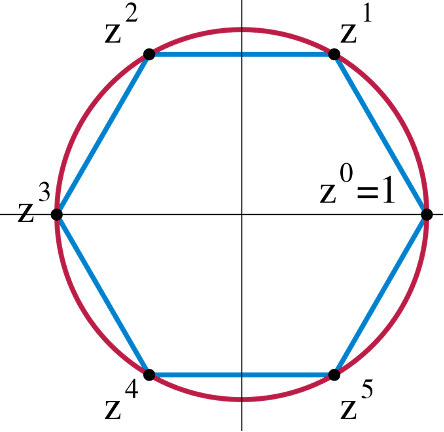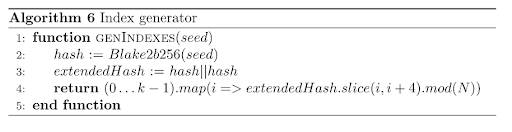# Autolykos Line-by-line#

Autolykos is a proof-of-work (PoW) algorithm utilized in blockchain mining. It's based on the principles outlined in the Equihash paper, which itself is an exploration of the generalized birthday problem. This guide provides an in-depth explanation of the Autolykos algorithm by breaking down its pseudocode and underlying mechanics.

## The Core Mechanism: An Overview#

In essence, miners are tasked with finding k (=32) elements from a set of N elements. The chosen elements should yield a sum that, when hashed, has a hash value lower than a target value. The first miner to solve this puzzle can add the next block to the blockchain.

## Autolykos Block Mining Pseudocode### Pre-Requisites: The Cyclic Group#

Before diving into the block mining procedure, it's important to understand that Autolykos requires:

• a very large cyclic group G
• of prime order q
• with fixed generator g
• And identity element e.

This prime group returns integers in Z/qZ during the Blake2b256-based hashing function.

Example cyclic group with generator z, identity element 1, order 6However, we won't dwell on the cyclic group as it plays a minor role in the overall PoW scheme.

## Line 1: Input h and m#

Input: upcoming block header hash m, block height h

The Autolykos Proof-of-Work (PoW) algorithm utilizes two key inputs to initiate its functioning:

• Block Height (h): Represents the height of the blockchain, or simply, the number of blocks.
• Upcoming Block Header Hash (m): This is a cryptographic hash of the components of the upcoming block header, which includes elements such as the previous block header hash, the Merkle root, a nonce, and other relevant data.

The block header hash is a hash of the block header components, (i,e previous block header hash, Merkle root, nonce, etc.)

## Algorithm 3: Hash Function#

### Preliminaries#

Before delving into the subsequent lines of the algorithm, it's crucial to understand the hash function denoted by H() in Autolykos. This function is called Algorithm 3 and is based on the Blake2b256 hash function.

#### Functionality#

The Algorithm 3 evaluates if the Blake2b256 hash of the given inputs is lower than $$2^{256}$$. If so, it returns the hash after a modulo operation with $$q$$, where $$q$$ is the prime order of group $$G$$. Should the hash exceed this value, the function iterates until a suitable hash is obtained. It should be noted that the outputs of Algorithm 3 are numeric hashes in the range Z/qZ.

### Line 2: Computation of List R#

In this line, the algorithm focuses on generating a list called $$R$$. This list is populated with numeric hashes called $$r$$ values. Each $$r$$ value is produced by the function $$\text{takeRight}(31, H(j \parallel h \parallel M))$$.

#### Variables Explained#

• $$j$$: An integer within the range $$[0, N)$$.
• $$h$$: Block height, as described earlier.
• $$M$$: A constant 8kb data segment used to slow down the hash calculation.

Here, $$\text{takeRight}(31, H(...))$$ essentially selects the 31 least significant bytes from the 32-byte output of the H(...) function, discarding the most significant byte.

For example, if $$j = 1$$, then $$r_1 = \text{takeRight}(31, H(1 \parallel h \parallel M))$$.

This operation is performed $$N-1$$ times to populate the list $$R$$ with $$N$$ elements, using an incrementing $$j$$.

## Lines 3/4: begin while loop and guessing#

Once the list $$R$$ is calculated, the algorithm enters a loop where it makes a nonce guess. The loop continues until a nonce is found that produces a hash less than a given target value.

Calculate r
while true do


## Lines 5/6: seed for generating indexes#

Line 5, i = takeRight(8, H(m||nonce)) mod N, produces an integer in [0,N). Algorithm 3 is utilised but with m and the nonce as inputs. Once the hash H(m||nonce) is returned, the eight least significant bytes are kept and passed through mod N. As a side note, the highest possible integer value with 8 bytes is 264 – 1, and assuming N = 226_, an 8-byte hash mod N will result in the first few digits being zero. The number of zeros in i decreases as N grows.

Line 6 produces e, a seed for index generating. Algorithm 3 is called with inputs i (generated in line 5), h, and M. Then, the most significant byte of the numeric hash is dropped, and the remaining 31 bytes are kept as value e. It should also be noted that value e can be retrieved from list R instead of being computed since e is an r value.

## Line 7: index generator#

Element index J is created using Algorithm 6 with inputs e, m,_ and nonce. Function genIndexes is a pseudorandom one-way that returns a list of k (=32) numbers in [0,N).

genIndexes functionA couple of extra steps are not shown in the pseudocode, such as a byteswap. The creation and application of genIndexes can be explained via the following example:

GenIndexes(e||m||nonce)...

• hash = Blake2b256(e||m||nonce) = [0xF963BAA1C0E8BF86, 0x317C0AFBA91C1F23, 0x56EC115FD3E46D89, 0x9817644ECA58EBFB]_
• hash64to32 = [0xC0E8BF86, 0xF963BAA1, 0xA91C1F23, 0x317C0AFB, 0xD3E46D89 0x56EC115F, 0xCA58EBFB, 0x9817644E]_
• extendedhash (i.e., byteswap and concatenate 4 bytes by repeating first 4 bytes) = [0x86BFE8C0, 0xA1BA63F9, 0x231F1CA9, 0xFB0A7C31, 0x896DE4D3, 0x5F11EC56, 0xFBEB58CA, 0x4E641798, 0x86BFE8C0]_

The following python code shows slicing the extended hash, returning k indexes. In this example we are assuming h < 614,400, thus N = 226 (67,108,864).

Slicing and mod N

for i in range(8):
idxs[i << 2] = r[i] % np.uint32(ItemCount)
idxs[(i << 2) + 1] = ((r[i] << np.uint32(8)) | (r[i + 1] >> np.uint32(24))) % np.uint32(ItemCount)
idxs[(i << 2) + 2] = ((r[i] << np.uint32(16)) | (r[i + 1] >> np.uint32(16))) % np.uint32(ItemCount)
idxs[(i << 2) + 3] = ((r[i] << np.uint32(24)) | (r[i + 1] >> np.uint32(8))) % np.uint32(ItemCount)


The main takeaway is that slicing returns k indexes which are pseudorandom values derived from the seed, i.e., e, m, and nonce.

return [0x2BFE8C0, 0x3E8C0A1, 0xC0A1BA, 0xA1BA63, 0x1BA63F9, 0x263F923, 0x3F9231F, 0x1231F1C, 0x31F1CA9, 0x31CA9FB, 0xA9FB0A, 0x1FB0A7C, 0x30A7C31, 0x27C3189, 0x31896D, 0x1896DE4, 0x16DE4D3, 0x1E4D35F, 0xD35F11, 0x35F11EC, 0x311EC56, 0x1EC56FB, 0x56FBEB, 0x2FBEB58, 0x3EB58CA, 0x358CA4E, 0xCA4E64, 0x24E6417, 0x2641798, 0x179886, 0x39886BF, 0x86BFE8]

This index can be translated to values in base ten as it refers to numbers in [0, N). For instance, 0x2BFE8C0 = 46131392, 0x3E8C0A1 = 65585313, 0xC0A1BA = 12624314, and so on. The miner uses these indexes to retrieve k r values.

The genIndexes function prevents optimisations as it is extremely difficult, basically impossible, to find a seed such that genIndexes(seed) returns desired indexes.

## Line 8: sum of r elements given k#

Using the index generated in line 7, the miner retrieves the corresponding k (=32) r values from list R and sums these values. This might sound confusing but let’s break it down.

Continuing the example above, the miner stores the following indexes:

{0 | 46,131,392},
{1 | 65,585,313},
{2 | 12,624,314},
{3 | 10,599,011},
…
{31 | 8,830,952}


Given the indexes above, the miner retrieves the following r values from list R stored in memory.

{0 | 46,131,392} → _dropMsb(H(46,131,392||h||M))_
{1 | 65,585,313} → _dropMsb(H(65,585,313||h||M))_
{2 | 12,624,314} → _dropMsb(H(12,624,314||h||M))_
{3 | 10,599,011} → _dropMsb(H(10,599,011||h||M))_
…
{31 | 8,830,952} → _dropMsb(H(8,830,952||h||M))_


Note that Takeright(31) operated on a 32-byte hash can also be written as dropMsb (drop most significant byte).

Since the miner already stores list R in RAM, the miner does not need to compute k (= 32) Blake2b256 functions and instead looks up the values.

This is a key feature of ASIC resistance. An ASIC with limited memory needs to compute 32 Blake2b256 iterations to get the values that could have been looked up in memory, and fetching from memory takes much less time.

An ASIC with limited memory would require 32 Blake2b256 instances physically on the die to achieve one hash per cycle, which would require more area and higher costs. It's simple to prove that storing list R in memory is well worth the trade-off.

Assume the following, a GPU has a hash rate of G = 100MH/s, N = 226, k = 32, block interval t = 120 seconds, and elements are looked up every four hashes. We can assume that elements are looked up every four hashes because, for each nonce guess, multiple elements such as i, J, and H(f) require Algorithm 3, i.e. blake2b hash, instances.

We can estimate that each r value will be used, on average, (G * k * t)/(N*4) = 1430.51 times.

Once the 32 r values are looked up, they are summed.

## Line 9 - 12: check if the hash of sum is below target#

The sum of the 32 r values is hashed using Algorithm 3, and if the output is below target b, the PoW is successful, m and nonce are returned to network nodes, and the miner is rewarded in ERG. If the sum hash is above the target, Lines 4 – 11 are repeated with a new nonce.

If you have made it this far, congratulations! After reading all of this information, you should have a good understanding of Autolykos v2! If you want a visual demonstration of Autolykos, please see the graphic at the end of this document. If you would like a video explanation, you can find it here.